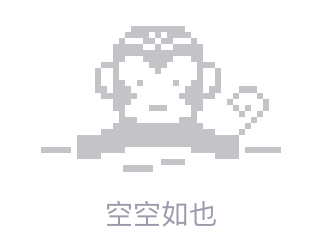# 以下合并排序算法有什么问题？

As the question states, I'm having trouble finding where is the issue within the following algorithm. It is the aux function for mergesort, i.e. the one used for combining sorted arrays.

``````func Merge(toSort *[]int, p, q, r int) {
arr := *toSort
L := arr[p:q]
R := arr[q:r+1]
fmt.Println(L)
fmt.Println(R)
i := 0
j := 0

for index := p; index <= r; index++ {
if i >= len(L) {
arr[index] = R[j]
j += 1
continue
} else if j >= len(R) {
arr[index] = L[i]
i += 1
continue
}

if L[i] > R[j] {
fmt.Println("right smaller")
arr[index] = R[j]
j += 1
continue
}
if L[i] <= R[j] {
fmt.Println("left smaller")
arr[index] = L[i]
i += 1
continue
}

}

}
``````

For `arr := []int{1,7,14,15,44,65,79,2,3,6,55,70}` it gives as output `[1 2 2 2 2 2 2 2 3 6 55 70]`.

The JavaScript equivalent for this function works as expected, but I don't know why it isn't working in `Go`

Thank you2014/03/28 00:49
• algorithm
• 点赞
• 收藏
• 回答
私信

#### 3个回复